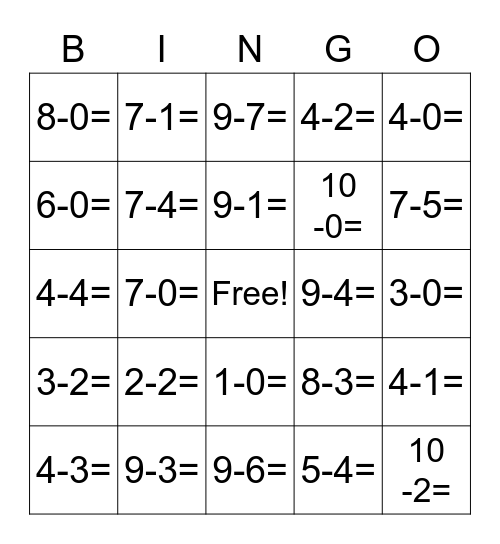# Subtraction Within 10 BINGOThis bingo card has a free space and 65 words: 1-1=, 2-1=, 3-1=, 4-1=, 5-1=, 6-1=, 7-1=, 8-1=, 9-1=, 10 -1=, 1-0=, 2-0=, 3-0=, 4-0=, 5-0=, 6-0=, 7-0=, 8-0=, 9-0=, 10 -0=, 2-2=, 3-2=, 4-2=, 5-2=, 6-2=, 7-2=, 8-2=, 9-2=, 10 -2=, 3-3=, 4-3=, 5-3=, 6-3=, 7-3=, 8-3=, 9-3=, 10 -3=, 4-4=, 5-4=, 6-4=, 7-4=, 8-4=, 9-4=, 10 -4=, 5-5=, 6-5=, 7-5=, 8-5=, 9-5=, 10 -5=, 6-6=, 7-6=, 8-6=, 9-6=, 10 -6=, 7-7=, 8-7=, 9-7=, 10 -7=, 8-8=, 9-8=, 10 -8=, 9-9=, 10 -9= and 10 -10=.

## Play Online

Share this URL with your players:For more control of your online game, create a clone of this card first.

## Probabilities

With players vying for a you'll have to call about __ items before someone wins. There's a __% chance that a lucky player would win after calling __ items.

Tip: If you want your game to last longer (on average), add more unique words/images to it.